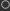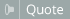Thread: Recommended MEMSET primer? View Single Post06-20-2018, 07:23 AM #6 mschnell Human being with feelings   Join Date: Jun 2013 Location: Germany Posts: 6,654I just did a test and what I said seems not to be correct. I found that 1<<31 is negative and 1<< 32 is zero. So eel seems to do logical operations with 32 bit even on reaper 64 bit But using multiplications, you seem to be able to store 52 bits in a slot: Code: ```descr: test1 @init x = 0; xf1 = 0; xf2 = 0; y = 0; z = 0; yf = 1; yfa = 1; loop(52, y = 1 << z; y != 0 ? ( x += 1; ); yf = yf * 2; yfa = yf + 1; yfb = yfa - 1; yf == yfb ? ( xf1 += 1; ); yf === yfb ? ( xf2 += 1; ); z += 1; );``` Try changing the 52 to e.g. 53-Michael __________________ www.boa-sorte.de